# Program to check Smith Number in Java

In this tutorial you will learn about the Program to check Smith Number in Java and its application with practical example.

In this tutorial, we will learn to create a Java Program to check Smith Number in Java using Java programming.

## Prerequisites

Before starting with this tutorial we assume that you are best aware of the following Java programming topics:

• Java Operators.
• Basic Input and Output function in Java.
• Class and Object in Java.
• Basic Java programming.
• If-else statements in Java.
• For loop in Java.
• User define functions.

## What is Smith Number?

A Smith number is a composite number(that can be formed by multiplying two smaller positive integers) in which the sum of its digits is equal to the sum of the digits of all its prime factors exclude 1.

Example “22” is a Smith Number as the sum of the digits of 22 are :2+2=4 .The prime factors of 22 are: 2,11 ( sum = 2+1+1 = 4). so 22 is Smith number.

## Program to check Smith Number in Java

In this program we will find given number is Smith Number or not. We would first declared and initialized the required variables. Next, we would prompt user to input the value. Later we will find Smith number.

## Output

Smith Number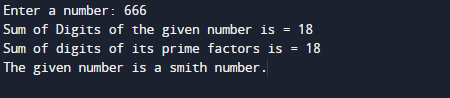Not a Smith number.In the above program, we have first declared and initialized a set variables required in the program.

After that take a value of number from user and store that number into variable “number”.as shown in image below.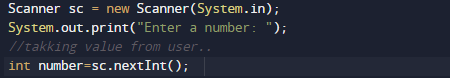## Logic to Find Smith Number

• Take a number from user and initialize to variable.
• And then we find the sum of its digits using “SumOfDigit()” user define Function.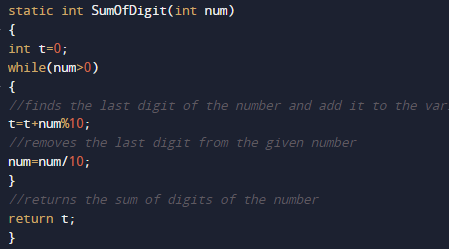• After that we will find prime factors of the given number in function Prime(int n).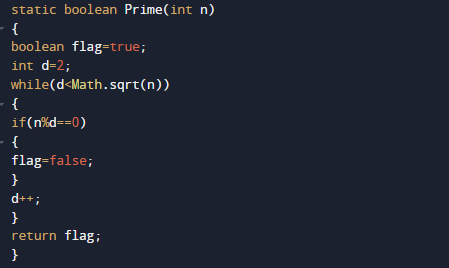• Now the sum of digits of its prime factors using “SumPrimFact(int no)”  .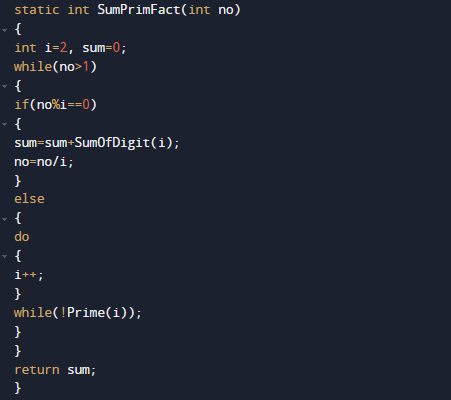• At the end we Compare the sum of digits with the sum of digits of prime factors.

If both are equal, then entered  number is a smith.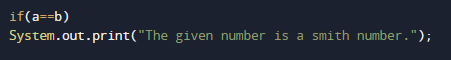else, not a smith number.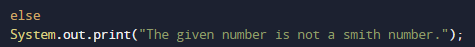so in that fashion we find given number is Smith number or not.

In this tutorial we have learn about the Program to check Smith Number in Java and its application with practical example. I hope you will like this tutorial.